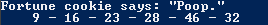# Assignment #56

## Code

```    /// Gian Adoremos
/// Period: 7
/// Program Name: FiftySixth Program
/// Date Finished: 11/19/2015

import java.util.Random;

{
public static void main(String[] args)
{
Random r = new Random();

int fortune = 1 + r.nextInt(6);
int fort1 = 1 + r.nextInt(54);
int fort2 = 1 + r.nextInt(54);
int fort3 = 1 + r.nextInt(54);
int fort4 = 1 + r.nextInt(54);
int fort5 = 1 + r.nextInt(54);
int fort6 = 1 + r.nextInt(54);

if (fortune == 1)
else if (fortune == 2)
else if (fortune == 3)
System.out.println("Fortune cookie says: \"Even More Poop.\"");
else if (fortune == 4)
else if (fortune == 5)